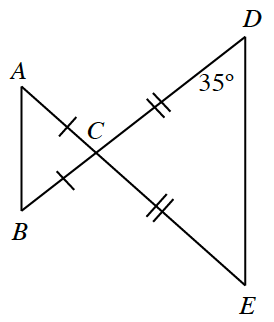### Home > CCG > Chapter 5 > Lesson 5.2.2 > Problem5-65

5-65.Copy the diagram at right onto your paper.

1. Find the measures of all the angles in the diagram.

Isosceles triangles have two congruent base angles.

Vertical angles are congruent. So $m∠ACB=m∠DCE$.

2. Make a flowchart showing that the triangles are similar.

As long as you have a minimum of two pairs of congruent angles you can prove the triangles similar by using $\text{AA}\sim$.

3. Cheri and Roberta noticed their similarity statements for part (b) were not the same. Cheri had stated $ΔABC∼ΔDEC$, while Roberta maintained that $ΔABC∼ΔEDC$. Who is correct? Or are they both correct? Explain your reasoning.

Cheri's similarity statement says the second triangle is a reflection and dilation of the first, while Roberta's statement says it is a rotation and dilation of the original triangle. Who is correct?